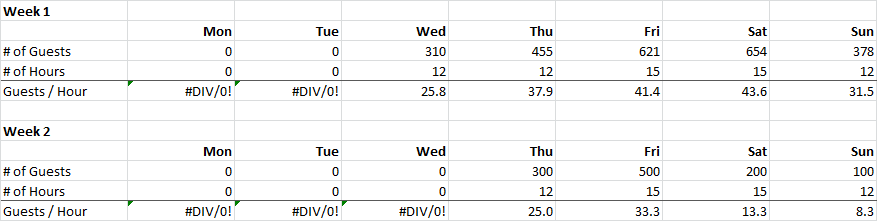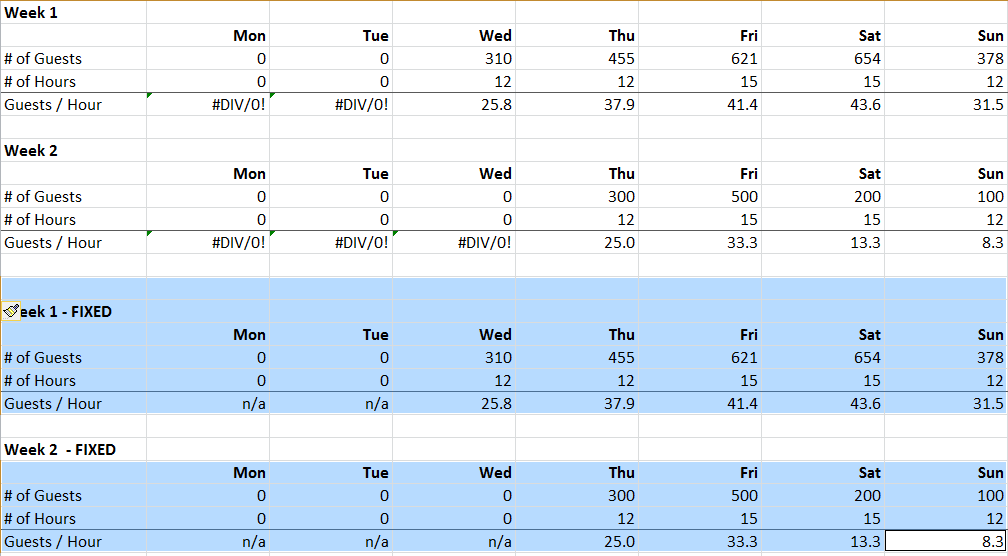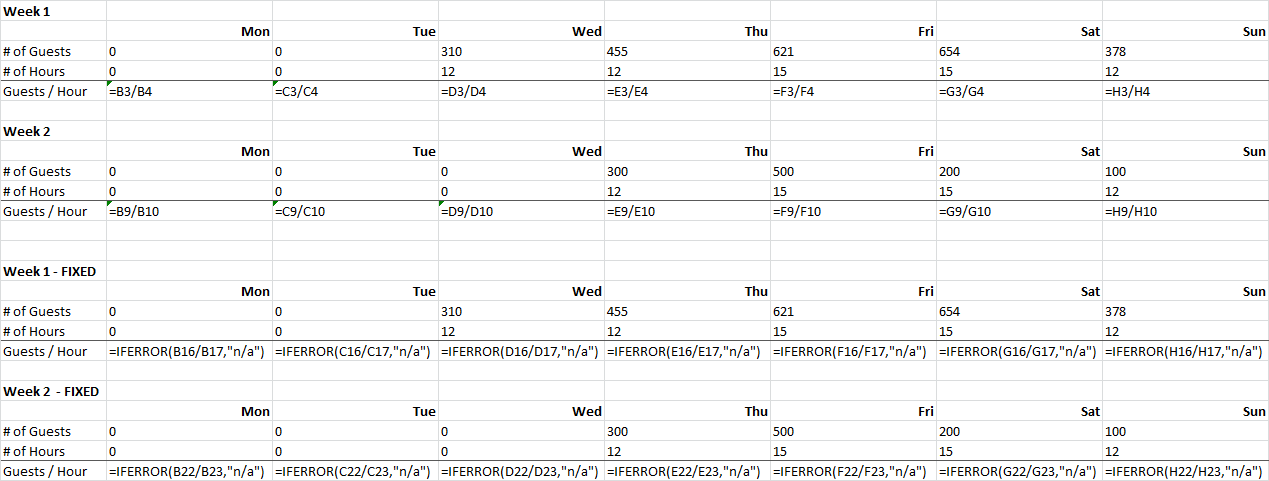# How to apply IFERROR on many cells automatically using Excel VBA Macro?

Sometimes you want to apply the “IFERROR” in Excel to trap errors and avoid “ugly” output, such as below:In this example, before applying IFERROR, you can see all the errors because of dividing by 0 for days that are not open.

Let’s say for a report to your manager, you may have cleaner output. Instead of showing “#DIV/0”, you want to show “n/a” as the data point is not available.

The best way is to use the “IFERROR” formula to detect an error, and substitute the error with a your desired message.

We would change the original formula from (showing “#DIV/0”):

`=B3/B4`

Into this (showing “n/a”):

`=IFERROR(B3/B4,"n/a")`

And this will be the desired result.This is the desired result. Where a cell shows “n/a” in the event there is an error.

But what if you have a lot of different formulas or tables where you want to apply IFERROR and do not want to do it manually? Below is a VBA macro that will do exactly that. This is a huge time saver for large, complex spreadsheets.

A few special functionality makes the script more user friendly:

• Only applies IFERROR to formula cells, and avoids empty cells and cells with a value (not formula)
• Avoids adding the IFERROR into a cell if it is done already (if the cell formula starts with “=IFERROR(“
• Works for multiple selection areas, so you can select as many parts of a spreadsheet as you need
• Debug messages using “Debug.Print” in case something breaks or you want to modify the script. (I have commented them out below.)

The scripts have three key components:

1. First loops determines if there are multiple selected area and if there is, script loops through each selected area
2. Script loops through each cell in a range for each selected area and calls the function that modifies the cell
3. The actual part of the script that modifies the cell and wrap the “IFERROR” around appropriate formulas.

```Sub IfErrorWrapSelectedCells()
Dim selectedRange As Range
Dim singleArea As Range
Set selectedRange = Application.Selection 'set all cell areas being selected

'work for multiple selected areas
If selectedRange.Areas.Count = 1 Then
loopThroughRange selectedRange
Else
For Each singleArea In selectedRange.Areas 'more than 1 selected area
loopThroughRange singleArea
Next
End If
End Sub

Function loopThroughRange(r As Range)

Dim c As Range
Dim activeRow As Range
Dim loopRow, loopCol As Integer

For Each c In r 'loop through each cell
WrapCell c 'wrap the cell
Next

End Function

Function WrapCell(c As Range)

Dim s As String
If (c.Formula = "") Then
'Debug.Print "Exit"
Exit Function 'if blank cell, exit
ElseIf (LCase(Left(c.Formula, 8)) = "=iferror") Then
Exit Function 'if already has iferror, exit
ElseIf (Left(c.Formula, 1) = "=" Or Left(c.FormulaR1C1, 1) = "+") Then
'Debug.Print "Apply" &  "=IFERROR(" & Right(c.Formula, Len(c.Formula) - 1) & ")"
s = "=IFERROR(" & Right(c.Formula, Len(c.Formula) - 1) & ",""n/a"")" 'Change the iferror fallback value here
c.Formula = s
Exit Function 'if formula, apply
Else
'Debug.Print "Not Formula"
'Debug.Print Left(c.FormulaR1C1, 1)
Exit Function 'Else not formula, exit
End If
End Function
```

Below is the end result. Hope you enjoy it.Result of running the script on the selected areas. Formula view shows that only cells with real formulas have IFERROR applied to it by the script above.